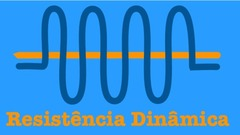RESISTÊNCIA DINÂMICA - The derivative of eigenvalues and Lyapunov exponentsDynamical Resistance Day

 The derivative of eigenvalues and Lyapunov exponents13 de Novembro | 11h00min | Radu Shagin (PUC-Valparaiso, Chile)

Title: The derivative of eigenvalues and Lyapunov exponents (video)

We will start considering the following example. If η is a simple eigenvalue for the matrix A∈GLd(R), then every matrix B in a neighborhood of A will also have a simple eigenvalue η(B) which is the continuation of η. A simple computation gives the derivatives of the map B↦logη(B).
This simple example will help us study the differentiability of Lyapunov exponents for diffeomorphisms with dominated splittings. If the diffeomorphism f has a dominated splitting TM=E⊕F and preserves a probability measure μ, the average (integrated) Lyapunov exponent of E with respect to f and μ is λ(E,f,μ)=∫Mlog∣det(Df|E)∣dμ.
Every diffeomorphism g near f preserving the same measure μ will also have a dominated splitting TM=Eg⊕Fg, and a corresponding Lyapunov exponent λ(Eg,g,μ). We will see that if the bundles E and F have some regularity, then the map g↦λ(Eg,g,μ) is differentiable, and we can get formulas for the derivatives. We will present some applications to the study of the regularity of the metric and topological entropy with respect to the map. This is joint work with Francisco Valenzuela and Carlos Vásquez.